# Abstract

A hierarchical finite element is developed for the free vibration analysis of a liquid in a rigid cylindrical tank with or without a free surface. It is a hierarchical quadrilateral element and has the advantage that the hierarchical mode number is allowed to vary independently of direction. Liquid behavior in tanks with large aspect ratios can therefore be solved very accurately by using a higher hierarchical mode number in the longer direction than in the shorter one. Furthermore, it is possible to idealize the liquid by using only one element. The solution can therefore be obtained to any desired degree of accuracy simply by increasing the hierarchical mode number. In this method, the liquid behavior is described by the displacements alone. The pressure and velocity potential are not considered as unknowns. The results are compared with other methods and show good agreement.

Keywords:
Hierarchical finite element method; liquid; cylindrical rigid tank; free vibration; free surface; sloshing; displacement based

# 1 INTRODUCTION

There are many cases of fluid structure interaction, such as sloshing in liquid storage structures and dams, and vibration of components of a nuclear reactor in a fluid. In most cases, it is difficult or impossible to obtain analytical solutions for coupled systems. Thus we must approach the solution by numerical methods based specifically on the finite element method.

In the added mass approach, a part of the mass of the fluid is added to the structural model along the interface between the two fields. This approach neglects the compressibility of the fluid. Several methods based on the added mass approach for the analysis of the liquid storage structures were proposed (Housner, 1957Housner, G., (1957). Dynamic Pressure on Accelerated Fluid Containers, Bulletin of the Seismological Society of America, 47, 15-35., ASCE, 1986ASCE, Standard, (1986). Seismic analysis of safety related nuclear structures and commentary on standard for seismic analysis of safety related nuclear structures. ASCE, New York.).

In the Eulerian approach (Haroun, 1983Haroun M. A., (1983). Vibration Studies and Tests of Liquid Storage Tanks. J. of Earth. Engng and Struc. Dyn., 11, 179-206., Haroun, 1984, Veletsos and al, 1990Veletsos, A.S., Tang, Y. and Tang, H., (1990). Soil-Structure Interaction Effects for Liquid Containing Storage Tanks. Proceedings of the Fourth U.S. National Conference on Earthquake Engineering, California., Balendra 1982Balendra, T., Ang, K. K., Paramasivam P. and Lee S. L., (1982). Seismic design of flexible cylindrical liquid storage tanks. J. of Earth Engng and Stru Dyn, 19, 473-496., Hughes et al, 1981Hughes, T. J. R., Liu W. K., and Zimmermann T. K., (1981). Lagrangian Eulerian finite element formulation for incompressible viscous flows, Comp. Meth. Appl. Mech. Engng., 29, 329-349., Soria and Casadei, 1997Soria A. and Casadei F. (1997). Arbitrary Lagrangian-Eulerian multicomponent compressible flow with fluid-structure interaction. International Journal for Numerical Methods in Fluids. 25(11), 1263-1284., Legay and al, 2006Legay A., Chessa J. and Belytschko, T., (2006). An Eulerian-Lagrangian method for fluid-structure interaction based on level sets. Comput. Methods Appl. Mech. Engrg., 195, 2070-2087,, Dunne, 2007Dunne T., (2007). Adaptive Finite Element Approximation of Fluid-Structure Interaction Based on Eulerian and Arbitrary Lagrangian-Eulerian Variational Formulations. PhD thesis, University of Heidelberg., Cottet and al, 2008Cottet G., Maitre E., and Milcent T., (2008). Eulerian formulation and level set models for incompressible fluid-structure interaction. ESAIM-Math. Model. Numer. Anal., 42 (3), 471-492., Rannacher and Richter, 2010Rannacher, R. and Richter, T. (2010). An adaptive finite element method for fluid-structure interaction problems based on a fully eulerian formulation. In H.J. Bungartz, M. Mehl, and M. Sch"afer, editors, Fluid-Structure Interaction II, Modelling, Simulation, Optimization, number 73 in Lecture notes in computational science and engineering, 159-192., Sanches and Coda, 2010Sanches, R. A. K. and Coda, H. B. (2010). Fluid-structure interaction using an arbitrary Lagrangian-Eulerian fluid solver coupled to a positional Lagrangian shell solver. Mecánica Computacional, 29, 1627-1647., He and Qiao, 2011He P. and Qiao R., (2011). A full-Eulerian solid level set method for simulation of fluid-structure interactions. Microfluidics and Nanofluidics, 11, 557-567., Wick, 2013Wick, T., (2013). Fully Eulerian fluid-structure interaction for time-dependent problems, Comput. Methods Appl. Mech. Eng. 255, 14-26.), the velocity potential, the pressure, or the velocity describes the behavior of the fluid, while the displacements represent the movements of the structure. The solution of the coupled system can then be obtained by solving the two systems separately, but by considering the effects of the interaction in an iterative way. The coupled system can also be solved as being only one system, but this leads to non-symmetrical matrix equations which require special solution techniques. Mixed Lagrangian-Eulerian methods were also developed to solve this type of coupled systems using a velocity based formulation (Hughes et al, 1981Hughes, T. J. R., Liu W. K., and Zimmermann T. K., (1981). Lagrangian Eulerian finite element formulation for incompressible viscous flows, Comp. Meth. Appl. Mech. Engng., 29, 329-349., Nomura and Hughes, 1992Nomura, T. and Hughes, T. J. R., (1992. An arbitrary Lagrangian Eulerian finite element method for interaction of fluid and a rigid body, Comp. Meth. Appl. Mech. Engng., 95, 115-138.).

In the Lagrangian approach, the fluid behavior is in general described by a displacement field (Shugar and Katona, 1975Shugar, T. A. and Katona, M. G., 1975. Development of finite element head injury model. Journal of Engng. Mech., Div. ASCE, 101, 223-239., Zienkiewicz and Bettess, 1978Zienkiewicz, O., and Bettess, P., (1978). Fluid-Structure Dynamic Interaction and Wave Forces. An introduction of Numerical Treatment. Inter. J. of Num. Meth. in Eng 13, 1-16., Hamdi and al, 1978Hamdi M., Ousset Y., and Verchery, G., (1978). A Displacement Method For the Analysis of Vibrations of Coupled Fluid-Structure Systems. Inter. J. for Num. Meth. in Engng, 13, 139-150., Akkas and al, 1979, Wilson and Khalvati, 1983Wilson, E. and Khalvati, M., (1983). Finite Elements For the Dynamic Analysis of Fluid-Solid Systems. International Journal of Numerical Methods in Engineering, 19, 1657-1668., Chen and, Taylor, 1990Chen HC, Taylor RL. (1990). Vibration analysis of fluid-solid systems using a finite element displacement formulation. Int J Numer Methods Eng. 29, 683-698., Idelson, 2003Idelson S., (2003). A Lagrangian meshless finite element method applied to fluid-structure interaction problems. Computers Structures. 81, (8-11), 655-671., Ryzhakov and al, 2010Ryzhakov P. B., Rossi R., Idelsohn S. R. and Oñate E. (2010). A monolithic Lagrangian approach for fluid-structure interaction problems. Computational Mechanics. 46, (6). 883-899., and Fuyin and al 2014FuYin G., Chong J., Yuan L. and KeJian S. (2014), Dynamic responses and damages of water-filled cylindrical shell subjected to explosion impact laterally. Latin American Journal of Solids and Structures. 11, 1924-1940.). The motion of the fluid and structure being both described by only one displacement field, this approach has the advantage of very easily satisfying the compatibility and equilibrium conditions along the interface. So, a fluid structure system in interaction with a complex geometry can be analyzed efficiently by this method.

Many displacement based fluid elements using the Lagrangian approach were proposed for viscous and non-viscous liquids, like those available in the computer codes ADINA and ANSYS. (Shugar and Katona, 1975Shugar, T. A. and Katona, M. G., 1975. Development of finite element head injury model. Journal of Engng. Mech., Div. ASCE, 101, 223-239.), developed a displacement based fluid element with four nodes using a solid element with a shear modulus equal to zero and a specific bulk modulus.

When a non-fine grid is used to model a fluid in a rigid cavity, parasitic modes at non zero frequencies appear as well as multiple modes with the same frequency. To solve this problem, (Chen and Taylor, 1990Chen HC, Taylor RL. (1990). Vibration analysis of fluid-solid systems using a finite element displacement formulation. Int J Numer Methods Eng. 29, 683-698.), used a projection of the mass matrix combined with a reduction of the stiffness matrix.

The liquid in this analysis is regarded as non-viscous, irrotational and incompressible. Such simplification allows displacements, pressures, or velocity potentials to be the field variables of the liquid. In this study, only the displacements will be taken as variables. This allows the fluid element to be easily incorporated into the available structural analysis programs.

The purpose of this work is to present a new displacement based bi-hierarchical finite element and to apply it to the free vibration analysis of a non-viscous, irrotational and incompressible liquid in a cylindrical rigid cavity with a free surface. This element coupled with other structural hierarchical finite elements such as that developed by (Ouissi and Houmat, 2009Ouissi, M.N. and Houmat, A. (2009), Non axisymmetric free vibration analysis of linearly varying thickness shells of revolution by a bi-hierarchical finite element. Latin American Journal of Solids and Structures, 6, (2). 105-129.) can easily idealize liquid structure interaction. Because of its hierarchical nature, only one element can idealize the whole liquid.

The hierarchical finite element method, also known as the p-version of the finite element method is more accurate and converges faster than the h-version. Indeed, when the exact solution is analytic everywhere the rate of convergence of the p-version is exponential, whereas that of the h-version is only algebraic. The quality of the solution is not very sensitive to the distortions of the elements, which allows the use of flattened elements or great ratio on sides without penalizing the accuracy too much. In addition, as a hierarchical formulation is adopted for the representation of displacements, the stiffness matrix relative to a given degree imbricates those of lower degrees. This makes it possible to obtain in an economical way a sequence of solutions instead of only one solution as it is the case of the h-version (Babuska and al., 1982Babuska I., Szabo B., (1982). On the rates of convergence of the finite element method. Int. J. Num. Meth. Engng., 18, 323-341.), (Szabo, 1990Szabo B.A. (1990). The use of a priori estimates in engineering computations. Comp. Meth. Appli. Mech. Engng., 82, 139-154.).

The h-p version of the finite element method possesses the features of both the p-version and the h-version. In this method, the accuracy is improved by increasing both the degree of the interpolating polynomial, and the number of elements (Szabo and al., 1991Szabo B.A. and Babuska I., Finite element analysis. John Wiley & Sons, (1991).).

The hierarchical shape functions are generally selected in the Serendipity space. In this paper, the shape functions are constructed from the shifted Legendre orthogonal polynomials. These shape functions were introduced by (Houmat, 2004Houmat A., (2004). Three dimensional hierarchical finite element free vibration analysis of annular sector plates. Journal of Sound and Vibrations. 276, 181-193.). They are different from those introduced by (Peano, 1975Peano A. G., 1975. Hierarchies of conforming finite elements, Ph.D. Thesis, Washington University.). Indeed, these polynomials are defined in the interval [0,1], whereas those of Peano are defined in the interval [-1,1].

# 2 GOVERNING EQUATION

The liquid motion is governed by Euler's equation:

(1)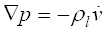Where p is the pressure, ∇ is a gradient operator ρl is the liquid density, and ν is the velocity vector.

In this formulation, velocity and pressure fields describe the motion of the liquid. Using the relationship between pressure and volume, one can write:

(2)Where u is the displacement vector and kl is the bulk modulus. Introducing the small amplitude motion assumption, Euler's equation is reduced to the acoustic wave equation:

(3)Where c is the acoustic speed given by:

(4)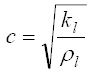In (Equation 3), only the pressure field describes the liquid motion. The same wave equation can be derived from the Navier's equation for an isotropic, homogeneous, and elastic medium as:

(5)Where, G is the shear modulus. For an inviscid liquid, the shear modulus equals zero. (Equation 5) becomes:

(6)A weak form of the above expression for a liquid region Ω subjected to natural boundary conditions and critical respectively on the boundaries Γp and Γf can be expressed as:

(7)Where p, ng is the gravity direction vector, n is the direction vector normal to corresponding boundary, g is the gravitational acceleration, Γl is the free surface, and ũ is a virtual displacement field. The second term in the left part of the above equation results from the free surface boundary condition relative to the sloshing movement.is the prescribed pressure on Γ

# 3 HIERARCHICAL FINITE ELEMENT FORMULATION

The liquid is discretized by four nodes hierarchical axisymmetric quadrilateral isoparametric finite elements (see Figure 1). All The liquid (inside and at the free surface) can be discretized into only one element if the geometry is varying linearly or if it is constant.

The radial, circumferential, and axial displacement components u, v, and w along the respective directions R, Z and ϑ may be written in the form

(8-a)(8-b)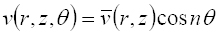(8-c)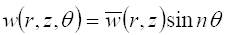Figure 1
Hierarchical axisymmetric liquid finite element

In the above, the dependence on time is removed by assuming that the displacements vary sinusoidally in phase at the same frequency. The displacements ū(r, z),(r, z), and(r, z) may be expressed in terms of the hierarchical finite element generalized displacements using appropriate shape functions.

## 3.1 Shape Functions Selection

They can be classified in three categories: nodal shape functions, side shape functions, and internal shape functions. The hierarchical shape functions for a one-dimensional element are:

(9)Where Pi (α) are the shifted Legendre orthogonal polynomials defined as:

(10)For C° continuous problems, the first two linear shape functions of the standard finite element method are retained. Higher order C° shape functions vanish at each end of the element. These are used to describe the displacements in the interior of the element.

The displacements ū(r, z),(r, z) for a quadrilateral hierarchical finite element can be expressed as(r, z), and

(11-a)(11-b)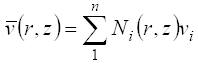(11-c)Where

(12)In the above, p and q denote the hierarchical mode numbers along ξ and η, respectively. The matrix form of (equations 11) is

(13)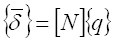Where

(14)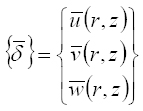(15)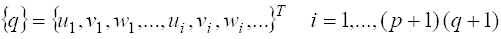(16)(17)Using the same shape functions matrix for the displacement fields u and ũ, one can write

(18)(19)Where {q}is the nodal displacement vector and {} is an arbitrary constant vector.

Using the variational form of (7) and replacing u and ũ by their expressions in (18) and (19), one can obtain the following matrix equation

(20)Where, [Kl ] is the stiffness matrix associated with the volumetric deformation, [Ks ] is the stiffness matrix associated to the sloshing, and {f} is the load vector. They are expressed as

(21)(22)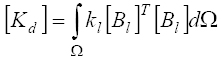(23)(24)Where kl is the liquid bulk modulus, Ω is the volume of the liquid, [N] is the shape function matrix, and [Bl ] is a matrix defined as

(25)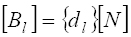## 3.2 Liquid Stiffness Matrix

The stiffness matrix is the superposition of the volumetric deformation stiffness matrix [Kd ] and the sloshing one [Ks ].

(26)### 3.2.1 Volumetric Deformation Stiffness Matrix [Kd]

The liquid deformation is given by

(27)Where {∇} is a differential operator defined as

(28)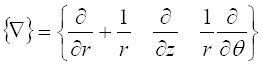Generalized displacements expressed in terms of the deformation are written as

(29)Where {df } is a differential operator defined as

(30)Substituting exp. (25) into (22), the element stiffness matrix can be written as

(31)Where k = 2 for n = 0 and k = 1 for n = 1, 2, ... (n: circumferential wave number)

### 3.2.2 Sloshing Stiffness Matrix

The vectors {n} and {ng} are orthogonal then, the sloshing stiffness matrix (23) can be written

(32)To evaluate the sloshing stiffness matrix [Ks ], the global cylindrical coordinates (r, z) must be expressed in terms of dimensionless local coordinates (ξ, η). A characteristic of the liquid element is that the surface local coordinate ξ varies from 0 to 1, while the local coordinated η remains constant and is equal to 1 because of its position at the free surface.

The final expression of the sloshing stiffness is

(33)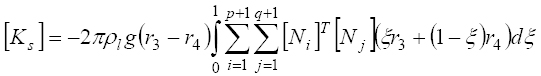As a result, the stiffness matrix, superposition of the deformation stiffness matrix and the sloshing one, is

(34)## 3.3 Liquid Mass Matrix

By replacing the matrix [N] by the expression (16), the liquid mass matrix can be written

(35)# 4 RESULTS AND DISCUSSIONS

The convergence and comparison studies must be carried out to ensure the reliability of the results. The results are given by the frequency parameter Ω which is expressed in terms of the vibration frequency ω by

(36)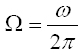## 4.1. Convergence and Validation

The effectiveness of the proposed liquid element was examined for the vibration of a liquid in a rigid circular cylindrical cavity and in a rigid circular cylindrical tank.

### 4.1.1 Rigid Circular Cylindrical Cavity

It is assumed that the cavity has a radius equal to 1 and a height equal to 1 as shown in Figure 2. The bulk modulus and the density are equal to 1. To validate the results, a standard FEM program has been elaborated, where 2100 axisymmetric liquid elements with four nodes were used. Because spurious modes cannot be eliminated, the real patterns will be recognized by plotting each mode.

Figure 2
Liquid in a cylindrical rigid cavity

Table 1 shows the convergence of the first six modes in terms of both of hierarchical mode numbers p and q following respectively the radial and axial directions for one and two elements. Considering the square shape of the cavity, the two hierarchical mode numbers will be equal.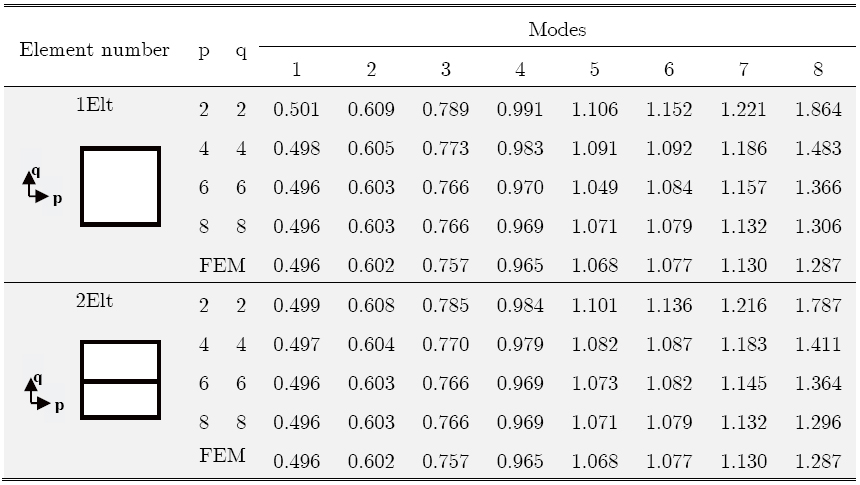Table 1 shows that for the two idealization one and two elements, an accuracy of two digits after the comma is reached for the first two modes for p=2 and q=2.

For modes 3, 4, 5; the convergence is reached for p=6 and q=6 for a one element model, and for p=4 and q=4 for a two elements model. The sixth mode reaches the convergence for p=8 and q=8 for one element, and p=6 and q=6 for two elements.

It is apparent that by increasing the number of elements, the hierarchical mode numbers p and q necessary to reach convergence are smaller. But the degrees of freedom number become more important. The matrices size is smaller if one increases p and q rather than the element number.

Figure 3 shows the eight independent modes of a four node liquid element. The first six modes are constant-strain modes and the other two are bending modes. One can find these two kind of modes for the bi-hierarchical liquid element.

Figure 3
Eight independent displacement modes of a four node liquid element

The first eight acoustic modes of the considered example are shown in figure 4. The first five modes are constant-strain modes and the other three are bending modes. These modes show the shift of a liquid relative to the walls while being in contact, which reflects the modeling of the interaction that is, imposing the same normal displacement to the sides. In this case, the normal displacement is equal to zero because the liquid is in a rigid cavity.

Figure 4
First Eight acoustic modes of a liquid in a cylindrical rigid cavity

### 4.1.2 Rigid Circular Cylindrical Tank

In order to verify its effectiveness, the hierarchical liquid element is used for the free vibration of a liquid with respectively a volumetric mass and a bulk modulus equals to 103 Kg/m3 and 2.068 109 KPa contained firstly in a rigid circular cylindrical tank of a radius equal to 6.48m for a height equal to 6.24m and secondly in a rigid circular cylindrical tank of a radius equal to 1.88m for a height equal to 4.75m (see figure 5).

Figure 5
Liquid in a rigid tank

The results are compared with those obtained by a standard FEM program where the axisymmetric liquid element with four nodes is used and with the theoretical frequencies of a liquid in a circular cylindrical storage tanks given by (Blevins, 1980)

(37)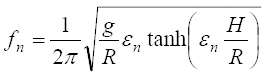Where g is the gravity acceleration, R is the radius of the cylinder, H is the liquid height and εn is the nth root of J(ε) Bessel function.

For the first example (Fig. 5.a), the comparison is carried out for one and two hierarchical elements with radial and axial hierarchical mode numbers varying from 2 to 8. The number of the axisymmetric liquid finite elements used in the standard FEM program is equal to 4212. The rigid tank of the second example (Fig. 5.b) being long, the comparison is carried out for only one hierarchical element with radial hierarchical mode number varying from 2 to 4 and axial hierarchical mode number varying from 2 to 8. The number of the axisymmetric liquid finite elements used in the standard FEM is equal to 2408.

Table 2 shows the convergence study for the first example (Fig. 5.a) of the first six modes with an increasing of the two hierarchical mode numbers p and q following respectively the radius and the axis directions for one and two elements. For the two idealization (Table 2), one and two elements, an accuracy of two digits after the comma is reached for the first mode with p=q=4. For the second mode, an accuracy of two digits is reached for p=q=8 with one element and for p=q=6 with two elements. For the modes 3, 4, 5; the convergence is reached for p=q=8 for the two idealization, but the matrices size is smaller if one increases p and q rather than the element number. For the second example, (Fig. 5.b) where the rigid tank is long, the convergence of the first six modes is given in Table 3. The liquid is idealized by only one element. The height of the liquid being greater than the tank radius, the increasing of the two hierarchical mode numbers p and q isn't the same. More the size is greater; more the increase of the hierarchical mode number is significant. As shown in Table 3, the hierarchical mode number q increase until 8, while p increase until 4. So, with this example, one can see that for different dimensions, it is not necessary to use the same hierarchical mode number for the radial and axial directions. This gives the advantage of having good results with smaller matrices and reduced computation time.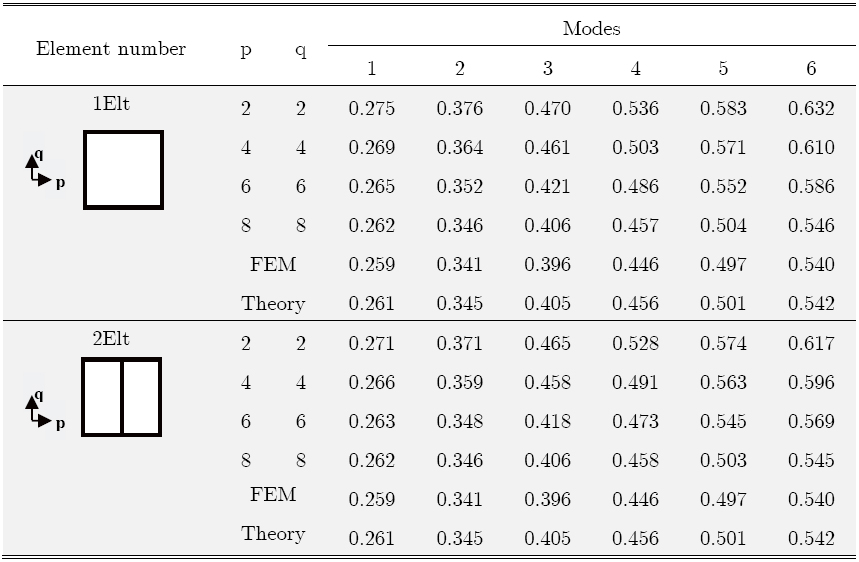Table 3
Convergence and frequency parameter comparison of a liquid in a rigid circular cylindrical tank (R =1.88, H=4.75).

## 4.2. Applications

The vibration analysis of a liquid in a rigid circular cylindrical tank is carried out (see figure 6). The radius and the liquid height are equal to 1m. The liquid volumetric mass and the bulk modulus are respectively equal to 103 Kg/m3 and 2.068 109 KPa.

Figure 6
Liquid in a rigid tank (H=1m, R=1m)

The liquid will be idealized by only one hierarchical finite element; indeed, its hierarchical nature provides this advantage. The height and the radius being equal, the radial and axial hierarchical mode numbers are equal to 8 (p=q=8).

Figures 7 and 8, shows respectively the first eight hierarchical sloshing modes and the first eight hierarchical acoustic modes. The liquid motion and then the deformed shapes are not the same by comparing the sloshing modes and the acoustic ones.

Figure 7
First Eight sloshing modes of a liquid in a cylindrical rigid tank

Figure 8
First Eight acoustic modes of a liquid in a cylindrical rigid tank

Indeed, for the first eight modes, the liquid moves and then deform only at the free surface, the liquid inside is almost steady state. This happens because at smaller frequencies, the liquid moves only at the free surface resulting in sloshing modes.

The first eight hierarchical sloshing modes vary from 0.664 to 1.549. The deformed shapes are the same as a beam vibration resting on a series of springs that is why there are used to model the free surface in some studies. The shape of the deformed is sinusoidal and the number of periods increases with the modes.

In the eight modes shown in figure 8, only the internal liquid deforms with high frequencies varying from 35.86 to 197.1 in the case studied. One can note the large gap with the sloshing frequencies. For these modes the liquid behaves as if it were in a rigid cavity.

The deformations are similar to those of acoustic modes of the example in Figure 2 shown in Figure 4. Then, these modes are called acoustic modes.

# 5 CONCLUSION

The hierarchical liquid finite element presented in this study is able to give accurate frequencies for liquids in axisymmetric cavities with or without free surface. The results show clearly that this element can be easily used for the analysis of the free vibration of a liquid in closed cavity or with a free surface. With this element, one is not constrained any more to have the same number of hierarchical modes in the two main directions (radial and axial) to idealize a liquid in a cavity or a tank which can be slender or lowered. Also, only one element can idealize a liquid in a rigid cavity (without a free surface) or a liquid in a rigid tank (with a free surface). Finally, this hierarchical finite element allows a triple increase in the accuracy, finite element number, and radial and axial hierarchical modes numbers.

# References

• ASCE, Standard, (1986). Seismic analysis of safety related nuclear structures and commentary on standard for seismic analysis of safety related nuclear structures. ASCE, New York.
• Babuska I., Szabo B., (1982). On the rates of convergence of the finite element method. Int. J. Num. Meth. Engng., 18, 323-341.
• Balendra, T., Ang, K. K., Paramasivam P. and Lee S. L., (1982). Seismic design of flexible cylindrical liquid storage tanks. J. of Earth Engng and Stru Dyn, 19, 473-496.
• Chen HC, Taylor RL. (1990). Vibration analysis of fluid-solid systems using a finite element displacement formulation. Int J Numer Methods Eng. 29, 683-698.
• Cottet G., Maitre E., and Milcent T., (2008). Eulerian formulation and level set models for incompressible fluid-structure interaction. ESAIM-Math. Model. Numer. Anal., 42 (3), 471-492.
• Dunne T., (2007). Adaptive Finite Element Approximation of Fluid-Structure Interaction Based on Eulerian and Arbitrary Lagrangian-Eulerian Variational Formulations. PhD thesis, University of Heidelberg.
• FuYin G., Chong J., Yuan L. and KeJian S. (2014), Dynamic responses and damages of water-filled cylindrical shell subjected to explosion impact laterally. Latin American Journal of Solids and Structures. 11, 1924-1940.
• Hamdi M., Ousset Y., and Verchery, G., (1978). A Displacement Method For the Analysis of Vibrations of Coupled Fluid-Structure Systems. Inter. J. for Num. Meth. in Engng, 13, 139-150.
• Haroun M. A., (1983). Vibration Studies and Tests of Liquid Storage Tanks. J. of Earth. Engng and Struc. Dyn., 11, 179-206.
• He P. and Qiao R., (2011). A full-Eulerian solid level set method for simulation of fluid-structure interactions. Microfluidics and Nanofluidics, 11, 557-567.
• Houmat A., (2004). Three dimensional hierarchical finite element free vibration analysis of annular sector plates. Journal of Sound and Vibrations. 276, 181-193.
• Housner, G., (1957). Dynamic Pressure on Accelerated Fluid Containers, Bulletin of the Seismological Society of America, 47, 15-35.
• Hughes, T. J. R., Liu W. K., and Zimmermann T. K., (1981). Lagrangian Eulerian finite element formulation for incompressible viscous flows, Comp. Meth. Appl. Mech. Engng., 29, 329-349.
• Idelson S., (2003). A Lagrangian meshless finite element method applied to fluid-structure interaction problems. Computers Structures. 81, (8-11), 655-671.
• Legay A., Chessa J. and Belytschko, T., (2006). An Eulerian-Lagrangian method for fluid-structure interaction based on level sets. Comput. Methods Appl. Mech. Engrg., 195, 2070-2087,
• Nomura, T. and Hughes, T. J. R., (1992. An arbitrary Lagrangian Eulerian finite element method for interaction of fluid and a rigid body, Comp. Meth. Appl. Mech. Engng., 95, 115-138.
• Ouissi, M.N. and Houmat, A. (2009), Non axisymmetric free vibration analysis of linearly varying thickness shells of revolution by a bi-hierarchical finite element. Latin American Journal of Solids and Structures, 6, (2). 105-129.
• Peano A. G., 1975. Hierarchies of conforming finite elements, Ph.D. Thesis, Washington University.
• Rannacher, R. and Richter, T. (2010). An adaptive finite element method for fluid-structure interaction problems based on a fully eulerian formulation. In H.J. Bungartz, M. Mehl, and M. Sch"afer, editors, Fluid-Structure Interaction II, Modelling, Simulation, Optimization, number 73 in Lecture notes in computational science and engineering, 159-192.
• Ryzhakov P. B., Rossi R., Idelsohn S. R. and Oñate E. (2010). A monolithic Lagrangian approach for fluid-structure interaction problems. Computational Mechanics. 46, (6). 883-899.
• Sanches, R. A. K. and Coda, H. B. (2010). Fluid-structure interaction using an arbitrary Lagrangian-Eulerian fluid solver coupled to a positional Lagrangian shell solver. Mecánica Computacional, 29, 1627-1647.
• Shugar, T. A. and Katona, M. G., 1975. Development of finite element head injury model. Journal of Engng. Mech., Div. ASCE, 101, 223-239.
• Soria A. and Casadei F. (1997). Arbitrary Lagrangian-Eulerian multicomponent compressible flow with fluid-structure interaction. International Journal for Numerical Methods in Fluids. 25(11), 1263-1284.
• Szabo B.A. (1990). The use of a priori estimates in engineering computations. Comp. Meth. Appli. Mech. Engng., 82, 139-154.
• Szabo B.A. and Babuska I., Finite element analysis. John Wiley & Sons, (1991).
• Veletsos, A.S., Tang, Y. and Tang, H., (1990). Soil-Structure Interaction Effects for Liquid Containing Storage Tanks. Proceedings of the Fourth U.S. National Conference on Earthquake Engineering, California.
• Wick, T., (2013). Fully Eulerian fluid-structure interaction for time-dependent problems, Comput. Methods Appl. Mech. Eng. 255, 14-26.
• Wilson, E. and Khalvati, M., (1983). Finite Elements For the Dynamic Analysis of Fluid-Solid Systems. International Journal of Numerical Methods in Engineering, 19, 1657-1668.
• Zienkiewicz, O., and Bettess, P., (1978). Fluid-Structure Dynamic Interaction and Wave Forces. An introduction of Numerical Treatment. Inter. J. of Num. Meth. in Eng 13, 1-16.

# Publication Dates

• Publication in this collection
July 2016